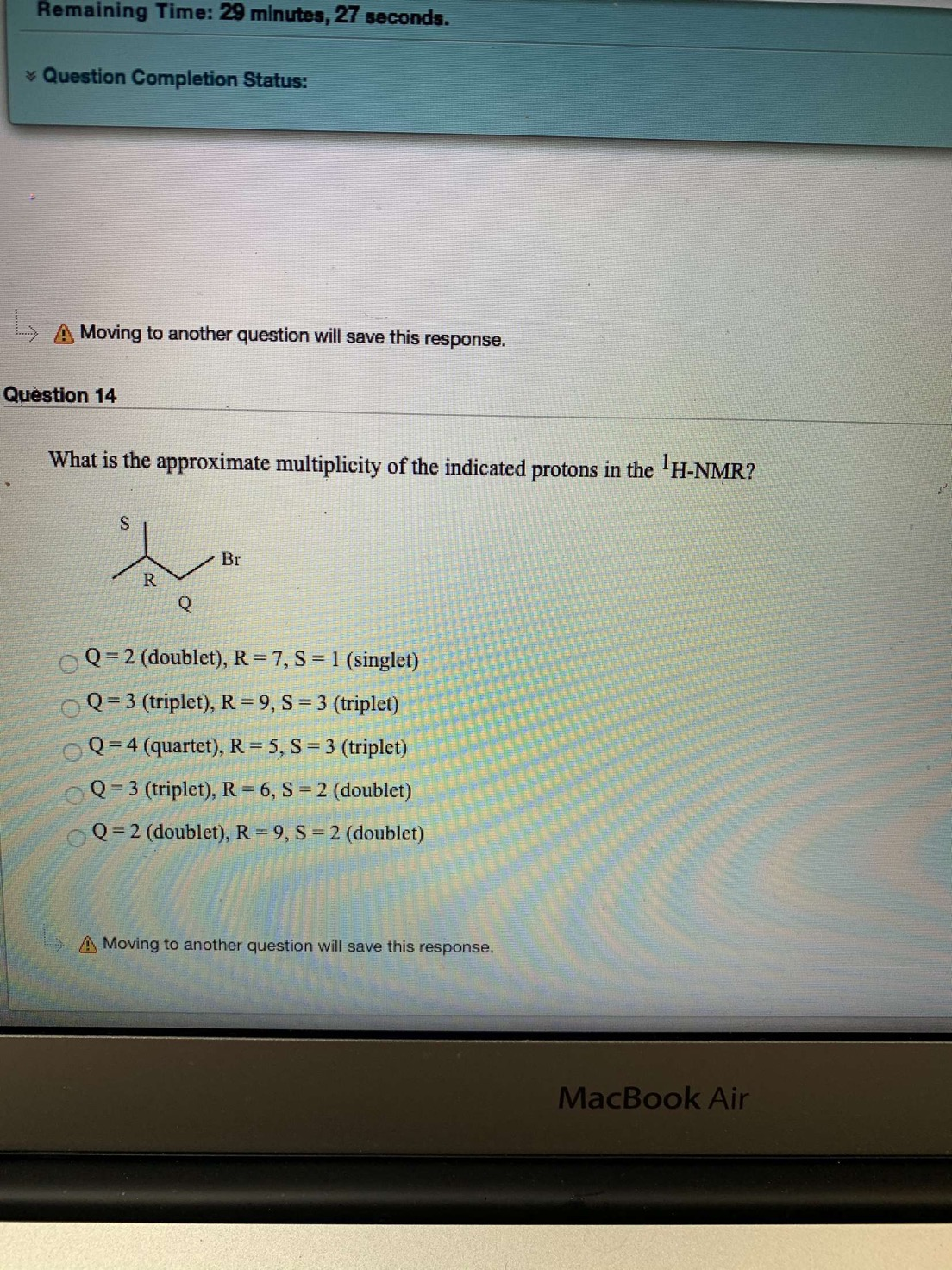# Remaining Time: 29 minutes, 27 seconds.Question Completion Status:A Moving to another question will save this response.Question 14What is the approximate multiplicity of the indicated protons in the H-NMR?BrRQQ 2 (doublet), R = 7, S = 1 (singlet)9, S = 3 ( triplet)Q 3 (triplet), RQ 4 (quartet), R= 5, S3 (triplet)Q 3 (triplet), R = 6, S2 (doublet)Q 2 (doublet), R = 9, S = 2 (doublet)A Moving to another question will save this response.MacBook Air

Question
1 viewshelp_outlineImage TranscriptioncloseRemaining Time: 29 minutes, 27 seconds. Question Completion Status: A Moving to another question will save this response. Question 14 What is the approximate multiplicity of the indicated protons in the H-NMR? Br R Q Q 2 (doublet), R = 7, S = 1 (singlet) 9, S = 3 ( triplet) Q 3 (triplet), R Q 4 (quartet), R= 5, S 3 (triplet) Q 3 (triplet), R = 6, S 2 (doublet) Q 2 (doublet), R = 9, S = 2 (doublet) A Moving to another question will save this response. MacBook Air fullscreen
check_circle

Step 1

In the NMR spectroscopy, the number of signals is give the information about the number of different environmental protons pr...

### Want to see the full answer?

See Solution

#### Want to see this answer and more?

Solutions are written by subject experts who are available 24/7. Questions are typically answered within 1 hour.*

See Solution
*Response times may vary by subject and question.
Tagged in

### Chemistry